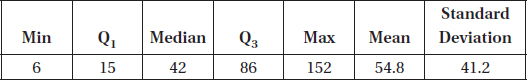# AP Statistics Multiple-Choice Practice Questions: Summarizing Distributions 2

### Test Information11 questions25 minutes

1. Suppose the average score on a national test is 500 with a standard deviation of 100. If each score is increased by 25, what are the new mean and standard deviation?

2. Suppose the average score on a national test is 500 with a standard deviation of 100. If each score is increased by 25%, what are the new mean and standard deviation?

3. If quartiles Q1 = 20 and Q3 = 30, which of the following must be true?

I.The median is 25.

II.The mean is between 20 and 30.

III.The standard deviation is at most 10.

4. A 1995 poll by the Program for International Policy asked respondents what percentage of the U.S. budget they thought went to foreign aid. The mean response was 18%, and the median was 15%. (The actual amount is less than 1%.) What do these responses indicate about the likely shape of the distribution of all the responses?

5. Data on the number of text messages sent one weekend by junior and senior high school students in a large high school is summarized as follows:An AP Statistics student checking the calculations finds that the message counts for all the students were underreported by 5. When the numbers are corrected, what are the corrected IQR and standard deviation?

6. Which of the following are true statements?

I.If the sample has variance zero, the variance of the population is also zero.

II.If the population has variance zero, the variance of the sample is also zero.

III.If the sample has variance zero, the sample mean and the sample median are equal.

7. When there are multiple gaps and clusters, which of the following is the best choice to give an overall picture of a distribution?

8. Suppose the starting salaries of a graduating class are as follows:

 Number of Students Starting Salary (\$) 10 15,000 17 20,000 25 25,000 38 30,000 27 35,000 21 40,000 12 45,000

What is the mean starting salary?

9. When a set of data has suspect outliers, which of the following are preferred measures of central tendency and of variability?

10. If the standard deviation of a set of observations is 0, you can conclude

11. A teacher is teaching two AP Statistics classes. On the final exam, the 20 students in the first class averaged 92 while the 25 students in the second class averaged only 83. If the teacher combines the classes, what will the average final exam score be?